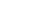#####Make your Weighing and Force test more accuracy
Home / All / load cell news,Industrial news /

February 25,2013

# How to select load cell (Weighing Sensor)?

The load cell selection should take into account many factors, the actual use of them we consider the following factors. In addition, the sensitivity of the load cell, the maximum number of divisions, the smallest verification scale value indicators must be considered in the sensor selection.
The number and range of the sensor
The choice of the number of sensors is based on the use of electronic weighing scale body needs to support the number of points (support points should the principles the scale body geometric center of gravity and the center of gravity coincides determined) may be. In general, the scale body several support points on the selection of a few sensors.
Sensor range selection can be based on the maximum weight value of the scale, the selection of the number of sensors, scale body weight, to determine the maximum partial load and dynamic load factor comprehensive evaluation. In general, the range of the sensor the closer is assigned to each sensor load, the higher weighing accuracy. However, in actual use, which scale body weight, tare weight, partial load and vibration impact loads, so the selection of the sensor, to consider a number of factors to ensure that the load sensor in addition to the object to be weighed, there the safety and life of the sensor. The empirical formula given below after a large number of experimental verification.
Formula is as follows:
C = K0 × K1 × K2 × K3 (Wmax W) / N
Where C rated range of a single sensor
W a scale body weight
The Wmax one called the maximum net weight of the object
N-scale body adopted by a number of support points
K0 insurance factor, generally between 1.2 to 1.3
K1 an impact coefficient
The K2 scale body's center of gravity offset coefficient
K3 a wind pressure coefficient
Accuracy class selection
The level of accuracy of the load cell sensor nonlinearity, creep, repeatability, hysteresis, sensitivity, and other technical indicators. Optional should not blind pursuit of high-grade sensors, should consider the level of accuracy and cost of electronic balance. Under normal circumstances, the total accuracy of the selection of the sensor is non-linear, non-repeatability and hysteresis of the three indicators and slightly higher than the rms value of the accuracy of the scale.
Posted by